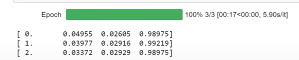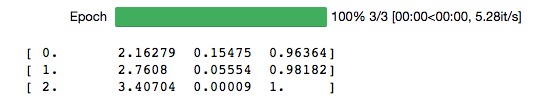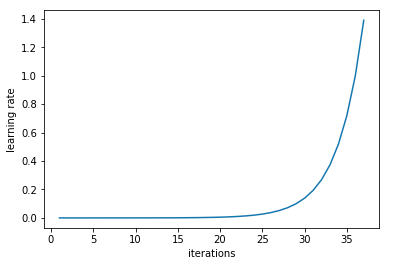# Determining when you are overfitting, underfitting, or just right?

(WG) #1

Overfitting if: training loss << validation loss

Underfitting if: training loss >> validation loss

Just right if training loss ~ validation loss

Question: How should we interpret >>, <<, and ~?

For example, what ratio between the training and validation loss would indicate that you are overfitting, underfitting, or in a good place?

13 Likes

(Sanjeev Bhalla) #2

Blockquote
Overfitting if: training loss >> validation loss
Underfitting if: training loss << validation loss

Arent you using << and >> wrongly?
I read first as training loss much greater than validation loss. That is underfitting.

I read second as training loss much less than validation loss. That is overfitting.

2 Likes

(WG) #3

You are right. Fixed.

0 Likes

(Sanjeev Bhalla) #4

Ok so your underlying question. How to interpret << >>

Not an expert but my assumptions have been

• Typically validation loss should be similar to but slightly higher than training loss. As long as validation loss is lower than or even equal to training loss one should keep doing more training.
• If training loss is reducing without increase in validation loss then again keep doing more training
• If validation loss starts increasing then it is time to stop
• If overall accuracy still not acceptable then review mistakes model is making and think of what can one change:
• More data? More / different data augmentations? Generative data?
• Different architecture?
4 Likes

(Jeremy Howard (Admin)) #5

Funnily enough, some over-fitting is nearly always a good thing. All that matters in the end is: is the validation loss as low as you can get it (and/or the val accuracy as high)? This often occurs when the training loss is quite a bit lower.

18 Likes

(Sudarsan Padmanabhan) #6

@jeremy
In lecture 1 videoThe difference between training and validation loss is the scale of 1/100 (around 0.01 - training 0.03 and validation 0.02). Is this like a metric that we should aim for when training different datasets?

For example when I trained baseball and cricket bats,the learning rate graph seems to matchthe loss function looks differentShould I try to get a graph similar to the one mentioned in the lecture?

0 Likes

(Stephan Rasp) #7

I have a question about the underfitting case where training loss > validation loss. I have seen this happen many times when training models but I don’t understand how this could happen. Why would the model ever perform better on the validation set than on the training set?

2 Likes

(Alan O'Donnell) #8

@raspstephan are you referring to seeing that while using the fast.ai lib? If I’m remembering right, that funny effect happens because of dropout: the training score is computed with dropout (which knocks out a bunch of the network, thereby weakening it), while the validation score is not (I suppose since it’s supposed to mimic how you’d perform on real test data, where we typically turn off dropout). Jeremy covers this oddity in lecture (assuming I’m not mis-remembering), I’ll try to find a link.

3 Likes

(Bhargav Kowshik) #9

From lesson 1 we have:

If you try training for more epochs, you’ll notice that we start to overfit, which means that our model is learning to recognize the specific images in the training set, rather than generalizing such that we also get good results on the validation set.

So, I took the 3 lines of code and ran for 50 epochs and got the following:

``````arch=resnet34
data = ImageClassifierData.from_paths(PATH, tfms=tfms_from_model(arch, sz))
learn = ConvLearner.pretrained(arch, data, precompute=True)

learn.fit(0.01, 50)
``````

Two things I observe from this graph about over-fitting are:

1. The training loss keeps decreasing after every epoch. Our model is learning to recognize the specific images in the training set.
2. The validation loss keeps increasing after every epoch. Our model is not generalizing well enough on the validation set.

### After 250 epochs

The trend is so clear with lots of epochs!

1 Like

(Khoa) #10

How do you plot both training loss and validation loss in 1 graph? @bkowshik

0 Likes

(Vishal R) #11

if you’re using matplotlib.pyplot:

``````import matplotlib.pyplot as plt
plt.figure()
plt.plot(train_losses)
plt.plot(validation_losses)
plt.show()
``````2 Likes

(Rafael Espericueta) #12

What do you make of this situation?

With more training, both training and validation loss are slowly increasing. It doesn’t look like typical overfitting. Is it underfitting?

0 Likes

(K Doherty) #13

learning rate too high?

0 Likes

(WG) #14

It looks like you’re over training u our network. Run lr finder to get your max lr and try training for around 10 epochs.

0 Likes

(Rafael Espericueta) #15

Is overfitting different than overtraining? With overfitting, the training error keeps going down while the test error goes up. In my situation they both are increasing?!

0 Likes

(Rafael Espericueta) #16

Have you seen this happen when the learning rate is too high?

0 Likes

(George Zhang) #17

Overtraining is what you do. Overfitting is what you got. The former is the process while the latter is the result.

If both training and validation losses are going up, as @mossCoder has pointed out, you are most likely using a learning rate too high.

The website below is a pretty good place to get some intuitions about the training process. I hope that you will enjoy it.

0 Likes

(Matt Ritter) #18

What if validation loss goes up for just one epoch and then keeps trending downward? Still time to stop?

0 Likes

(Vishal R) #19

I came up with a idea for an algorithm that can identify whether a model us overfitting, underfitting or is trained good enough using the loss values without visualizing them. Can someone contribute some interesting losses values they’ve encountered (preferably as a pickle object) with a small description so that I can test it out?

0 Likes